Function Repository Resource:

# HeatIndex

Get the human-perceived temperature using air temperature and relative humidity

Contributed by: Claudio Lobo Chaib Filho
 ResourceFunction["HeatIndex"][] gives the heat index for the user's location. ResourceFunction["HeatIndex"][loc] gives the heat index for a location loc. ResourceFunction["HeatIndex"][{t,rh}] gives the heat index for a temperature t and a relative humidity rh. ResourceFunction["HeatIndex"][…,form] gives the result in the desired output form.

## Details and Options

Heat index is the temperature perceived by humans, in the shade, taking into account air temperature and relative humidity. The function works with values between 80 °F and 110 °F for the temperature and values between 0% and 100% for relative humidity.
The function can be used with one or two arguments. If the function is used with one argument, the argument is the location (in the form of an Entity, GeoPosition or Here argument) or a list with air temperature and relative humidity information, where the user is requesting the heat index value. This information comes from the nearest weather station to that location and the value may vary over time.
In the case with two arguments, the second argument specifies the format of the output and can be a temperature, plot or an association giving both temperature and plot:
 "Temperature" value of temperature that is the heat index "Plot" plot of the curve of temperatures "PropertyAssociation" all results as an association
The plot shows the temperature curve (the x axis is the air temperature and y axis is the heat index temperature) for a given relative humidity.
Temperatures accepted are Quantity objects of temperature type such as "DegreesCelsius" or as a numeric value, in which case it is interpreted as Fahrenheit.
If the relative humidity unit is not specified in the rh argument, a value between 1 and 100 is considered as a percentage. If the value is given between 0 and 1, it is considered as a fraction and automatically converted into a percentage. For example, 0.25, 25 and Quantity[25,"Percent"] are all equivalent.
HeatIndex accepts the option "ResultUnit" to specify the unit of the result. The accepted values are "DegreesFahrenheit", "DegreesCelsius", "DegreesRankine" and "Kelvins". By default, the unit is inherited from the inputs. If no unit is provided, "DegreesFahrenheit" is used.

## Examples

### Basic Examples (4)

Get the current heat index at your location:

 In:=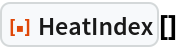Out=Get the heat index in Miami:

 In:=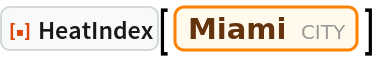Out=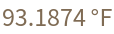Specify the desired output as "Temperature":

 In:=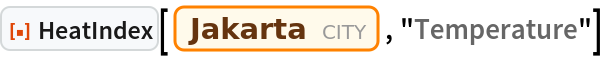Out=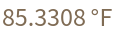Specify a temperature and humidity instead of a location:

 In:=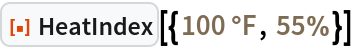Out=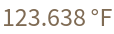### Scope (6)

The output unit, in this case degrees Celsius, is inherited from the input:

 In:=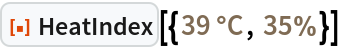Out=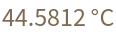If the temperature is not explicitly given with the unit, is interpreted as Fahrenheit (95 °F):

 In:=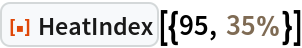Out=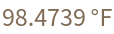If the relative humidity is not given as a Quantity, values between zero and one are interpreted as a fraction:

 In:=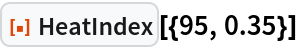Out=Humidity values between one and one hundred are interpreted as a percentage:

 In:=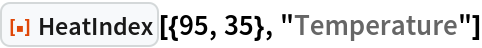Out=Create a plot of the heat index as temperature varies for relative humidity fixed at the current value of Cairo. The red point indicates the current temperature:

 In:=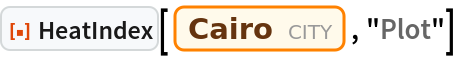Out=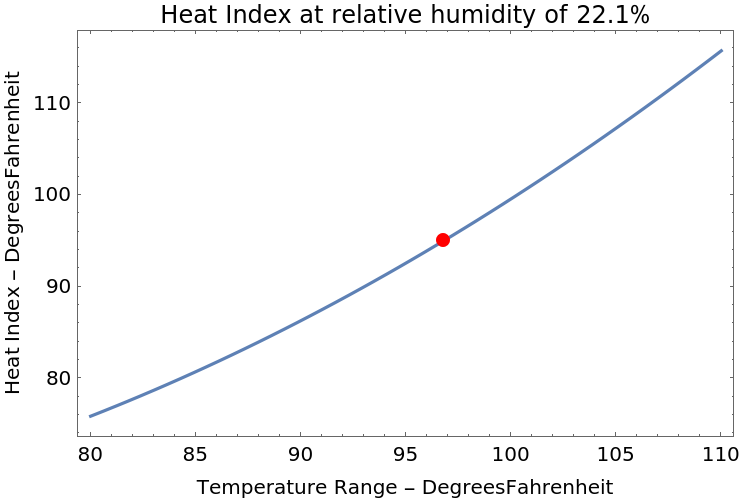Use "PropertyAssociation" to get the heat index and plot together:

 In:=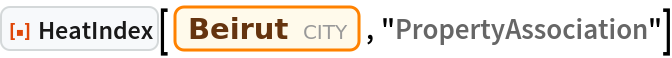Out=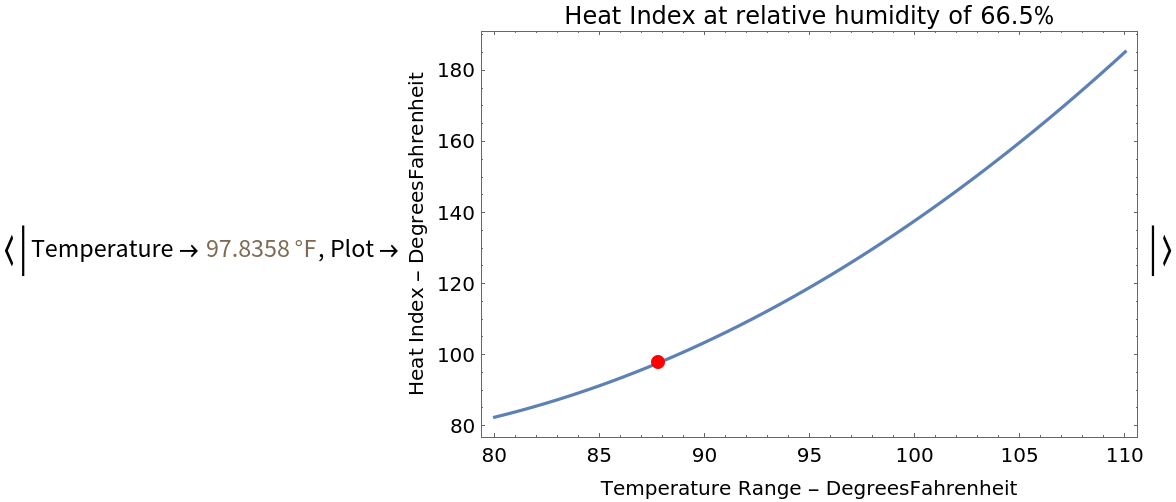Provide a GeoPosition instead of an Entity:

 In:=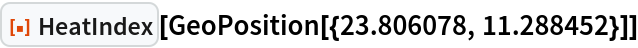Out=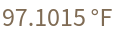### Options (2)

The "ResultUnit" option specifies the temperature unit for the result:

 In:=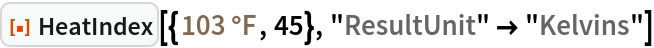Out=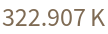Create a plot with the heat index values shown in Celsius:

 In:=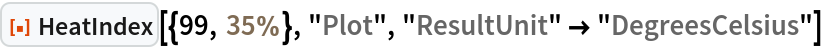Out=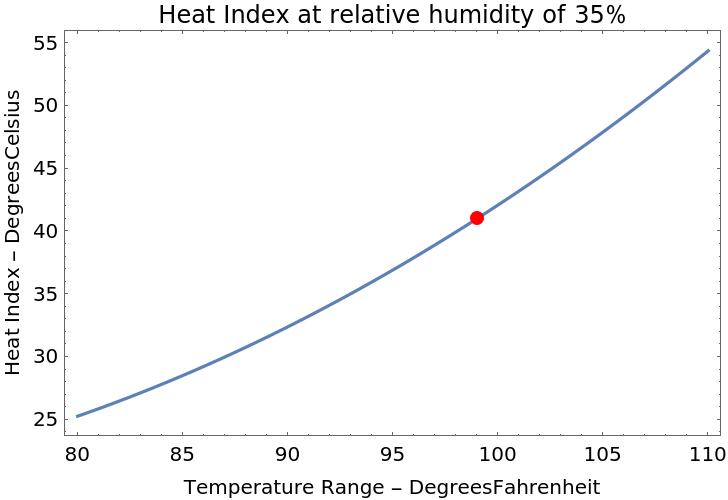### Possible Issues (4)

Values outside the supported range are inaccurate, and in these cases the result is a TildeTilde object:

 In:=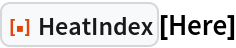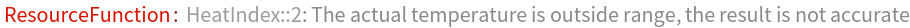Out=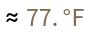Sometimes the nearest weather station of a specific location does not have data of air temperature and/or relative humidity:

 In:=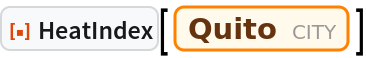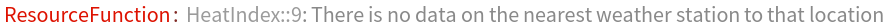Even if the unit of the input temperature is different from Fahrenheit, the x axis in the plot remains in Fahrenheit:

 In:=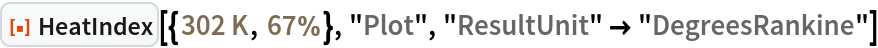Out=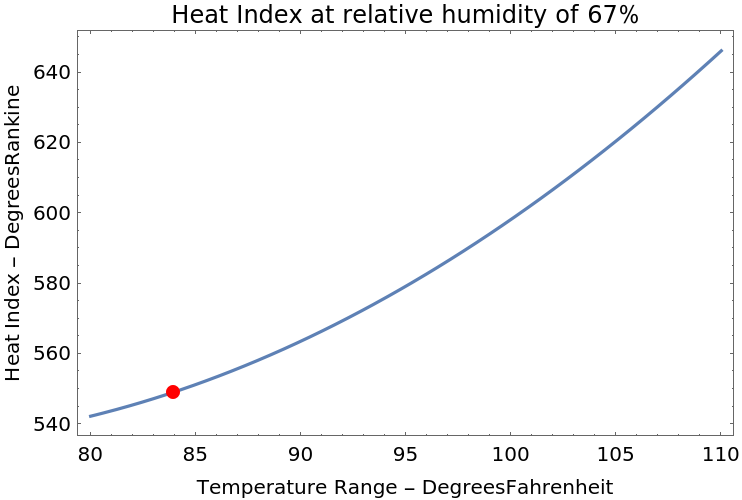For relative humidity values between 0% and 1%, the input must be explicitly given with a percentage unit. Otherwise, in the example below, 12% would be interpreted instead of 0.12%:

 In:=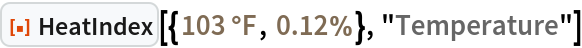Out=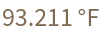## Publisher

Claudio Lobo Chaib Filho

## Version History

• 1.0.0 – 01 September 2020